##1. Desargues Theorem

For the triangles ABC, A'B'C', the two propositions are equivalent. The validity of one implies the validity of the other.
1) AA', BB', CC' lines pass all three through a point O (called perspectivity center or perspector).
2) The intersection points of the sides (prolongations) A''=(BC, B'C'), B''=(CA,C'A'), C''=(AB,A'B') lie on a line e (called perspectivity axis or perspectrix).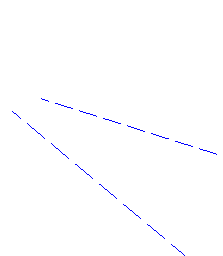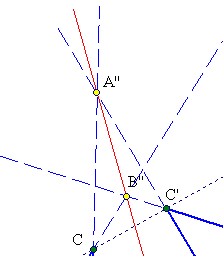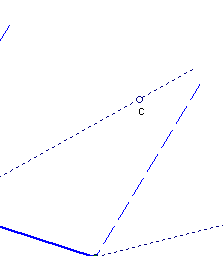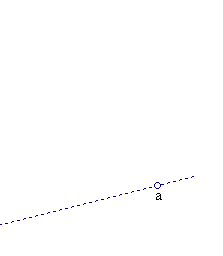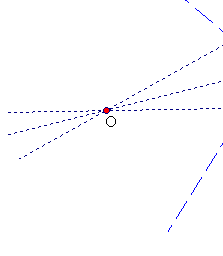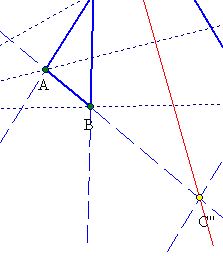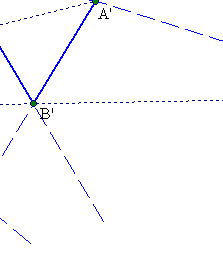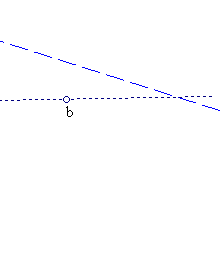To prove 1) => 2) apply Menelaus (see Menelaus.html ) three times to the triangles:
(a) OAB and line [C''A'B'] => (C''A/C''B)(A'O/A'A)(B'B/B'O) = 1,
(b) OAC and line [B''A'C'] => (B''C/B''A)(C'O/C'C)(A'A/A'O) = 1,
(c) OBC and line [A''C'B'] => (A''B/A''C)(B'O/B'B)(C'C/C'O) = 1.
Multiplying the sides of the equations: (C''A/C''B)(B''C/B''A)(A''B/A''C) = 1, which by Menelaus means that A'', B'', C'' are collinear points on the sides of triangle ABC.

To prove 2) =>1) consider triangles C''BB' and B''C'C which by the current hypothesis are perspective at A''. Hence by the proved part of the theorem corresponding side-pairs intersections O= (BB', CC'), A=(BC'', CB'') and A'= (B''C', C''B') are on a line, proving that ABC and A'B'C' are point perspective with respect to O.
See the file Duality.html for another kind of proof of 2) => 1) based on the duality of projective spaces.

##2. Corollary and applications

Let a variable triangle ABC have its vertices {B,C} on two fixed lines OB' and OC' respectively and its sides passing through three points {A'',B'',C''} lying on a fixed line. Then its third vertex A varies on a line OA' passing through O.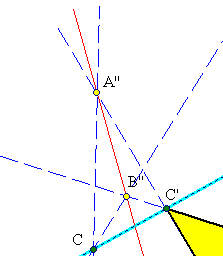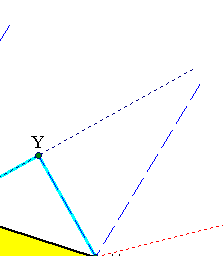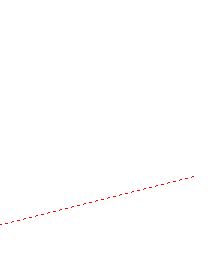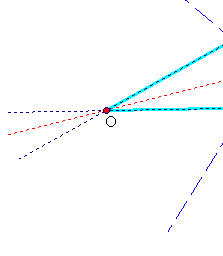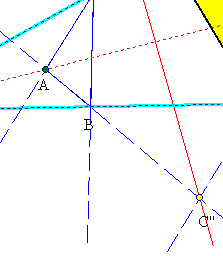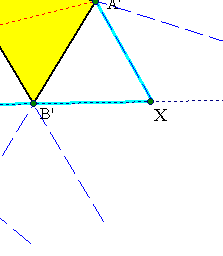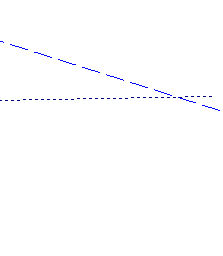Problem Given triangle OXY, inscribe to it a triangle A'B'C', such that its sides pass through three given points {A'',B'',C''} lying on a given line.

According to the corollary all triangles A'B'C' having their vertices {B',C'} on lines {OX,OY} respectively and their sides passing through the fixed points {A'',B'',C''} have their third vertex A' varying on a fixed line OA. Its intersection with the third side XY of the given triangle determines point A' of the desired triangle. This determines also completely triangle A'B'C', since its sides must pass through the given points {A'',B'',C''}.

Remark-1 A special case of the corollary occurs when line carrying {A'',B'',C''} is the line at infinity. This means that the variable triangle A'B'C' has sides always parallel to three given directions. In this special case one can see immediately, without using Desargues argument, that the third point varies on a line. Using this special case one can even reduce to it the general Desargues theorem by defining a projective map that sends the line carrying {A'',B'',C''} to the line at infinity.

Remark-2 Applying the corollary one can easily show inductively its generalization:
When a polygon varies so that its n sides pass through n fixed points lying on a given line and (n-1) of its vertices lie on (n-1) given fixed lines, then its n-th vertex describes also a line.

Remark-3 It is essential that points {A'',B'',C''} through which pass the sides of the variable triangle in the corollary are collinear. In the more general case in which these points are not collinear the locus of A is a conic. This is handled in the file Maclaurin.html . There is also a special case, in which these three points are not collinear, but line B''C'' passes through O and the locus is again a line. This case is handled below.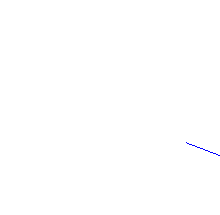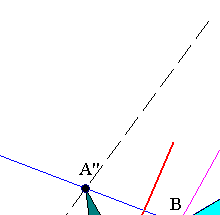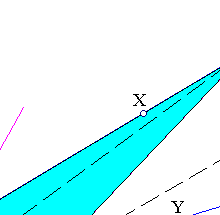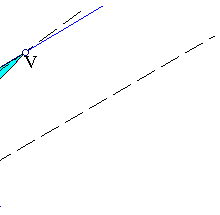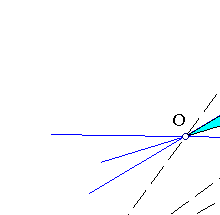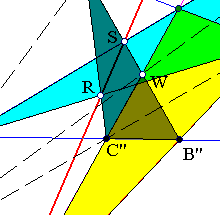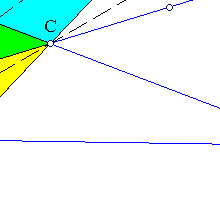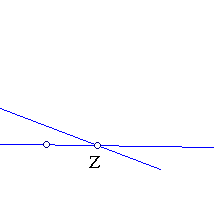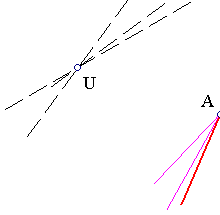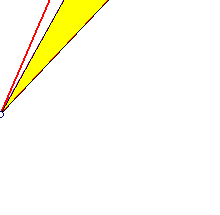##3. Application of homogeneous coordinates

Problem Let a variable triangle ABC have its vertices {B,C} on two fixed lines OX and OY respectively and its sides passing through three points {A'',B'',C''} with line B''C'' passing through O. Then its third vertex A varies on the line passing through points {R,S}, which are the intersection points respectively of the line-pairs (A''C'',OY) and (A''B'',OX).

To prove this consider the intersection points {V,W} of line-pairs correspondingly (AB'',OB) and (A''B'',C''B). Use a projective base in which A''(1,0,0), B''(0,1,0), C''(0,0,1) and calculate the coordinates of A:
Let lines {OX, OY, A''BC} be respectively of the form
OX: ax+by+cz=0,
OY: a'x+b'y+c'z=0,
A''BC: uy+vz=0.
Points {B,C} have then coordinates
(bv-cu, -av, au),
(b'v-c'u, -a'v, a'u).
Lines {BC'',CB''} have respectively the form:
(-av)x + (cu-bv)y=0,
(-a'u)x + (b'v-c'u)z=0.
Their intersection is point A with coordinates:
A: ((cu-bv)(b'v-c'u), av(b'v-c'u), a'u(cu-bv)).
Since lines {OX,OX} intersect at a point of line x=0, we find that b'=sb, c'=sc for a constant s.
Thus, the coordinates of point A become:
(-s(cu-bv)2, -sav(cu-bv), a'u(cu-bv)), which is simplifies to (s(cu-bv), sav, -a'u). (*)
On the other side the coordinates of points {S,R} are easily calculated to be
S: (-b,a,0) and R: (-sc,0,a'). The determinant of the coordinates of these two and (*) is then: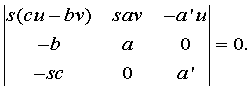This proves the collinearity of points {A,S,R}. By Desargues theorem triangles A''WC'' and OCV, being line-perspective, are also point-perspective with respect to a point U. One could start from this and show the collinearity of three points {A,S,R}. The calculation though seems to be slightly more complicated than the one given above.